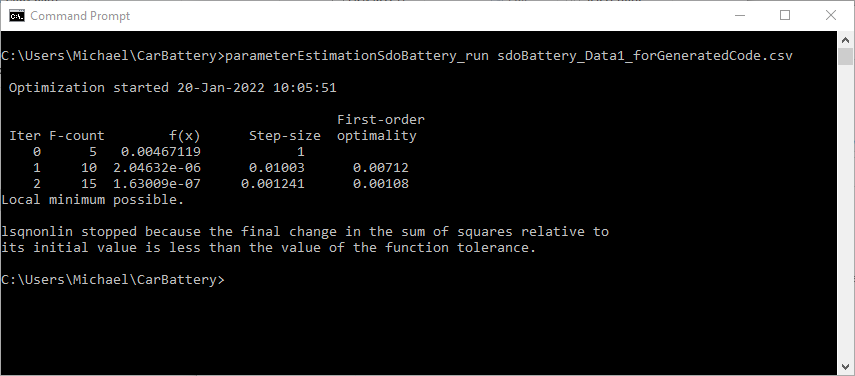# Generate MATLAB Code for Deployed Parameter Estimation Problems (GUI)

This example shows how to automatically generate MATLAB® code to solve a deployed parameter estimation problem. You use the Parameter Estimator to define an estimation problem for a battery and generate MATLAB code to solve this estimation problem in deployed mode using Simulink Compiler.

### Parameter Estimation for Battery Model

The "Deployed Application of Parameter Estimation" openExample('sldo/BatteryDegradationParameterEstimationDeploymentExample') example shows how to use Parameter Estimator to estimate parameters for a battery in an electric vehicle. In this example, you load a pre-configured Parameter Estimator session based on that example. Open the battery model.

`open_system('sdoBattery')`

Open Parameter Estimator app with a preconfigured session for parameter estimation.

```load sdoBattery_spesession_forDeployment spetool(SDOSessionData)```### Generate MATLAB Code

From the Estimate button, select Generate Functions for Deployment. This generates two MATLAB functions which are added to the MATLAB editor.The generated code is added to the MATLAB editor as two unsaved MATLAB functions. The first is a "setup" function.The "setup" function sets up the parameter estimation problem and prepares it to be solved in deployed mode. Significant portions of the function are:

• Specify Model Parameters to Estimate - Defines the model parameters being estimated.

• Define the Estimation Experiments - Specifies the measured data, and the signals they are associated with in the model. After parameter estimation, the model output should be close to the measured data.

• Create a Simulator - Creates a simulator for running the model to compare whether its output is similar to the measured data in the experiments.

• Prepare for Deployment - Prepares the experiments and simulator for deployment, for parameter estimation in deployed mode with Simulink Compiler. Saves these prepared objects to a MATLAB data file.

Select Save from the MATLAB editor to save the generated "setup" function.

The second generated function is a "run" function.The "run" function can be compiled to a stand-alone executable to be used in deployed mode with Simulink Compiler. Significant portions of the code in the "run" function are:

• Load Objects - Load the objects that were prepared for deployment by the "setup" function.

• Update Experiments - Update the experiments with new data. You will probably want to modify this portion of the code to suit the format of your data.

• Create Estimation Objective Function - Create an anonymous function that calls the subfunction `sdoBattery_optFcn`, which evaluates the model using each experiment and compares simulation and measured experiment outputs. This anonymous function is called by `sdo.optimize` at each iteration of the optimization problem to solve the estimation problem.

• Estimate the Parameters - Solve the estimation problem using the `sdo.optimize` command.

Select Save from the MATLAB editor to save the generated "run" function.

### Run Generated Code

Execute the setup file. This prepares the parameter estimation problem and saves prepared objects into the file `sdoBatteryObjectsToDeploy.mat`. Then you can run `parameterEstimationSdoBattery_run.m` in an environment with Simulink Compiler.Execute the "run" file. This can be compiled to a stand-alone executable using `mcc`, and run in deployed mode using Simulink Compiler.### Modify the Generated Code

You can modify the "setup" function to:

• Estimate different parameters, or specify different lower/upper bounds

• Include new experiments

You can modify the "run" function to:

• Modify the update-data portion to suit your data format.

• Change the options, such as the optimization solver, tolerances, etc.